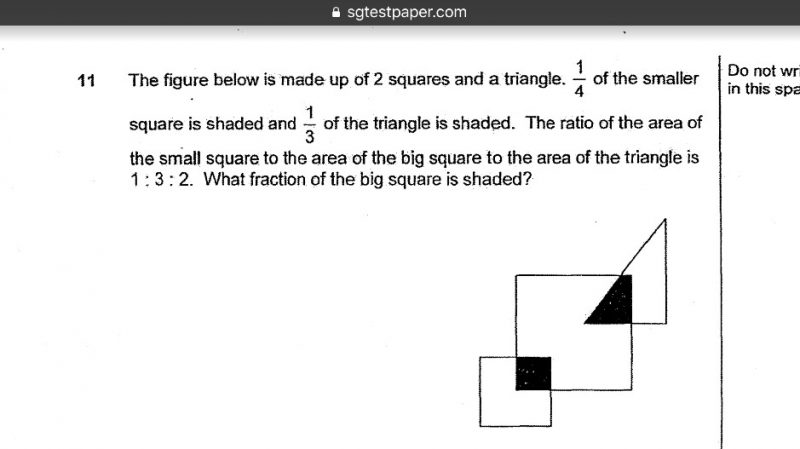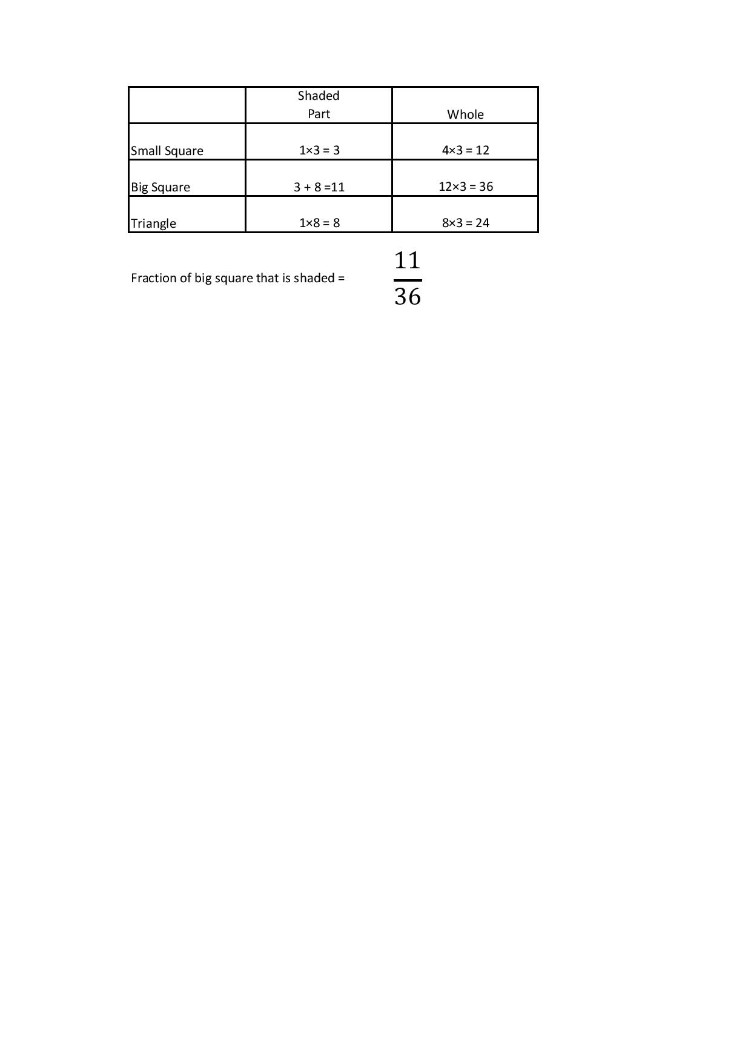# QuestionTia for detailed soln

1 Answer

# AnswerRatio of the small square to the big square to the triangle = 1 : 3 : 2

Since 1/4 of the small square is shaded, multiply the ratio above by 4.

Area of small square to big square to triangle = 4 : 12 : 8

The LCM of 3 and 8 is 24. So 1/3 = 8/24

Multiply the other ratios accordingly by 3 to maintain the equivalent ratios.

Then the shaded part of the big square comes from the small square and the triangle.

0 Replies 0 Likes